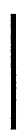DESCRIPTION   AND   OPERATION   OF INPUT   TRANSFER   ASSEMBLY
Custom SearchCHAP  2,  SEC  I DESCRIPTION   AND   OPERATION   OF PAR  9 INPUT   TRANSFER   ASSEMBLY fold-out   2)   supports   the   turbine   assembly in   the   converter   cover   assembly   68. .   L o c k u p    C l u t c h    C o m p o n e n t s    ( f i g. 3 7 3 ,    f o l d - o u t    2 ) .    T h e    l o c k u p    c l u t c h    i n - cludes   converter   cover   assembly   68,   piston 74,   clutch   plate   77,   reaction   plate   78   and related   minor   components.   Cover   assembly 68   serves   jointly   as   the   input   drive   for   the torque   converter   as   well   as   a   cylinder   for lockup   piston   74.   Clutch   plate   77   is   faced on   both   sides   with   a   friction   material.   It has   splines   on   its   internal   diameter   which engage   splines   on   the   hub   of   turbine   79. Reaction   plate   78   is   a   steel   ring   which includes   one   clutch   friction   surface.   Its o u t e r    d i a m e t e r    i s    h e l d    b e t w e e n    p u mp a s s e m b l y    8 2    a n d    c o v e r    a s s e m b l y    6 8    by bolts  86  and  nuts  71. c.   Operation   of   Torque   Converter and   Lockup   Clutch ( 1 )    T h e    t o r q u e    c o n v e r t e r    a nd lockup   clutch   are   combined   in   a   compact s u b a s s e m b l y    w h i c h    s e r v e s    a s    t h e    i n p ut member   for   the   main   transmission   section. This   combination   acts   as   a   torque   multi- plier,   a   fluid   coupling   or   a   direct-drive coupling    —   depending   upon   the   require- ment   at   any   particular   time. (2)   Engine   torque   is   delivered   to converter   cover   69   by   transfer   gear   67. Converter   cover,   lockup   clutch   piston   74, reaction   plate   78   and   converter   pump   as- sembly   82   rotate   as   a   unit   and   constitute the   drive   members.   Stator   assembly   94   is l o c a t e d    b e t w e e n    p u m p    a s s e m b l y    8 2    a nd t u r b i n e    a s s e m b l y    7 9 .    T h e    s t a t o r    i s    t he r e a c t i o n    m e m b e r .    T u r b i n e    a s s e m b l y    79 and   lockup   clutch   plate   77   rotate   as   a   unit and   with   converter   turbine   shaft   2   (fig. 375,    fold-out    4).    This    group    constitutes the   driven   members. (3)   The   cavity   within   the   assem- bled   components   is   kept   charged   with   oil w h i c h    c o n t i n u a l l y    c i r c u l a t e s    f o r    c o o l i ng and   lubrication.   Rotation   of   the   converter pump   while   the   vehicle   engine   is   at   idle s p e e d    w i l l    n o t    t r a n s m i t    a    s i g n i f i c a n t amount   of   power   hydraulically   to   the   con- verter   turbine.   Thus,   during   engine   idle, the   converter   acts   as   a   disengaged   clutch. The   pressure   of   the   oil   in   the   converter c a v i t y    k e e p s    t h e    l o c k u p    c l u t c h    p i s t on p u s h e d    t o    i t s    r e l e a s e d    p o s i t i o n    e x c e pt when   a   higher   pressure   is   applied   to   the opposite   side   of   the   piston.   This   occurs only   at   higher   speeds. (4)   When   the   engine   is   accelera- t e d ,    t h e    v a n e s    o f    t h e    c o n v e r t e r    p u mp throw   oil   radially   outward   and   into   the blades   of   the   turbine.   The   impact   of   this oil   against   the   turbine   blades   causes   the turbine   to   rotate.   Oil   leaves   the   vanes   of the  turbine  at  a  point  near  the  turbine  hub but   flowing   in   a   direction   opposite   to   con- verter   rotation.   This   flow   in   an   opposite d i r e c t i o n    i s    m o s t    p r o n o u n c e d    a t    l o w e r turbine   speeds.   At   higher   turbine   speeds, the   flow   changes   and   is   more   toward   the direction   of   rotation. ( 5 )    T o    r e t u r n    t o    t h e    c o n v e r t er pump,   the   oil   must   pass   between   the   stator v a n e s .    A t    l o w    t u r b i n e    s p e e d s ,    t h e    o il strikes   the   concave   sides   of   the   stator vanes.   This   tends   to   drive   the   stator   in   a direction   opposite   to   converter   rotation. The   stator   locks   up,   however,   and   the   sta- tor   blades   turn   the   flow   of   oil   and   direct it   in   the   same   direction   as   the   converter pump   rotates.   The   unexpended   energy   of the   oil   is   thus   added   to   the   movement   of the   pump.   This   is   the   key   to   torque   mul- tiplication. ( 6 )     W h e n     t u r b i n e     s p e e d preaches   pump   speed,   the   direction   of   oil flow   from   turbine   to   pump   changes.   The returning   oil   strikes   the   convex   sides   of the   stator   vanes,   causing   the   stator   to f r e e w h e e l .    U n d e r    t h e s e    c o n d i t i o n s ,    t he torque   converter   acts   as   a   fluid   coupling. There   is   no   multiplication   of   torque. ( 7 )    W h e n    t h e    c o n v e r t e r    r e a c h es sufficient   speed,   and   there   is   no   further need   for   either   torque   multiplication   or fluid   drive,   automatic   valves   direct   pres- sure   to   the   lockup   clutch   piston.   The   pis- ton   compresses   the   lockup   clutch   plate against   the   back   plate,   locking   the   con- verter   turbine   to   the   converter   cover   as- sembly. T h e r e f o r e ,    d u r i n g    l o c k u p ,    t he converter   turbine   is   driven   directly   from the   engine.   There   is   no   hydraulic   action within   the   torque   converter   because   the pump   and   turbine   rotate   as   a   unit.   The stator   freewheels   to   prevent   any   hydraulic drag.   There   is   no   loss   from   slippage,   which 12 Change   2Integrated Publishing, Inc. - A (SDVOSB) Service Disabled Veteran Owned Small Business